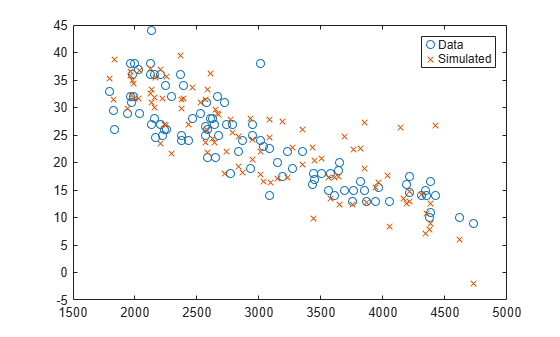# random

Simulate responses for nonlinear regression model

## Syntax

``ysim = random(mdl)``
``ysim = random(mdl,Xnew)``
``ysim = random(mdl,Xnew,'Weights',W)``

## Description

````ysim = random(mdl)` simulates responses from the fitted nonlinear model `mdl` at the original design points.```

example

````ysim = random(mdl,Xnew)` simulates responses from the fitted nonlinear model `mdl` to the data in `Xnew`, adding random noise.```
````ysim = random(mdl,Xnew,'Weights',W)` simulates responses using the observation weights, `W`.```

## Examples

collapse all

Create a nonlinear model of car mileage as a function of weight, and simulate the response.

Create an exponential model of car mileage as a function of weight from the `carsmall` data. Scale the weight by a factor of 1000 so all the variables are roughly equal in size.

```load carsmall X = Weight; y = MPG; modelfun = 'y ~ b1 + b2*exp(-b3*x/1000)'; beta0 = [1 1 1]; mdl = fitnlm(X,y,modelfun,beta0);```

Create simulated responses to the data.

```Xnew = X; ysim = random(mdl,Xnew);```

Plot the original responses and the simulated responses to see how they differ.

```plot(X,y,'o',X,ysim,'x') legend('Data','Simulated')```## Input Arguments

collapse all

Nonlinear regression model object, specified as a `NonLinearModel` object created by using `fitnlm`.

Vector of real, positive value weights or a function handle.

• If you specify a vector, then it must have the same number of elements as the number of observations (or rows) in `Xnew`.

• If you specify a function handle, the function must accept a vector of predicted response values as input, and returns a vector of real positive weights as output.

Given weights, `W`, `random` estimates the error variance at observation `i` by `MSE*(1/W(i))`, where MSE is the mean squared error.

New predictor input values, specified as a table, dataset array, or matrix. Each row of `Xnew` corresponds to one observation, and each column corresponds to one variable.

• If `Xnew` is a table or dataset array, it must contain predictors that have the same predictor names as in the `PredictorNames` property of `mdl`.

• If `Xnew` is a matrix, it must have the same number of variables (columns) in the same order as the predictor input used to create `mdl`. Note that `Xnew` must also contain any predictor variables that are not used as predictors in the fitted model. Also, all variables used in creating `mdl` must be numeric. To treat numerical predictors as categorical, identify the predictors using the `'CategoricalVars'` name-value pair argument when you create `mdl`.

Data Types: `single` | `double` | `table`

## Output Arguments

collapse all

Simulated response value, returned as a numeric vector. The simulated value is the predicted response values at `Xnew` perturbed by random noise. The noise is independent and normally distributed, with mean equal to zero and variance equal to the estimated error variance of the model.

## Alternatives

For predictions without added noise, use `predict`.

## Version History

Introduced in R2012a